# Akpan

Akpan spent 3/8 of his time in school during the week. What fraction of his time does he spend at home during the week?

Correct result:

h =  0.625

#### Solution:

$h=1-\frac{3}{8}=\frac{5}{8}=0.625$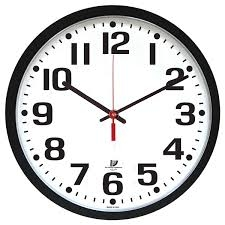We would be pleased if you find an error in the word problem, spelling mistakes, or inaccuracies and send it to us. Thank you!Tips to related online calculators
Need help calculate sum, simplify or multiply fractions? Try our fraction calculator.
Do you want to convert time units like minutes to seconds?

## Next similar math problems:

• HusseinHussein owns 450000 crowns (local currency). He spent at the bookstore 2 over 9 to buy some books and tales. He paid 3 over 5 of his money to buy his math book. a. Calculate the remaining amount of money with Hussein? b. Hussein lost 3 over 4 of the remai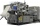Erika’s career consists of filing, typing and answering phones. She spends 1/6 of her time filing and 5/8 of her time typing. What fraction of her time does she spend answering phone calls?
• Mixed numbersRewrite mixed numbers so the fractions have the same denominator: 5 1/5 - 2 2/3
• EurosPeter spent a quarter of his pocket saving. He has 9 euros now in his wallet . How many euros spent?
• Product of two fractionsProduct of two fractions is 9 3/5 . If one of the fraction is 9 3/7. Find the other fraction.
• Fraction to decimalWrite the fraction 3/22 as a decimal.
• A quotientWhat is the quotient of 3/10 divided by 2/4 as a fraction?
• Cake fractions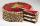Thomas ate 1/3 of cake, Bohouš of the rest of the cake ate 2/5. What fraction of cake left over for others?
• Jansen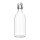Jansen had 1.3 litres of fruit juice. He drank 0.5 litres during lunch. How many litres of fruit juice does he have left?
• Hours 3How many hours are in 3 2/3 days?
• Doug bikedDoug biked 5 1/4 miles in 3/4 of an hour. What is his average speed?
• Pounds3 pounds subtract 1/3 of a pound.
• Outdoor temperatureThe outdoor temperature was 8 degrees at midnight. The temperature declined 5 degrees during each of the next 3 hours. What was the temperature at 3am?
• Minutes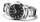Write as fraction in basic form which part of the week is 980 minutes.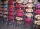Cris had 15000 . He spent 1/3 of his money on a table and the remaining on 5 similar chairs that cost the same. How much did one chair cost?Tom was asked to guess a fraction. The sum of 1/2 the numerator and 1/3 of its denominator is 30. If Tom subtracts 36 from its denominator, the fraction becomes 1/3. What is the fraction that Tom was asked to guess? (Leave your answer in simplest form)Simplify mixed numerals expression: 8 1/4- 3 2/5 - (2 1/3 - 1/4) Show your solution.